Courses

# Test : Perimeter And Area - 1

## 10 Questions MCQ Test Mathematics for Class 5 (V) - CBSE and NCERT Curriculum | Test : Perimeter And Area - 1

Description
This mock test of Test : Perimeter And Area - 1 for Class 5 helps you for every Class 5 entrance exam. This contains 10 Multiple Choice Questions for Class 5 Test : Perimeter And Area - 1 (mcq) to study with solutions a complete question bank. The solved questions answers in this Test : Perimeter And Area - 1 quiz give you a good mix of easy questions and tough questions. Class 5 students definitely take this Test : Perimeter And Area - 1 exercise for a better result in the exam. You can find other Test : Perimeter And Area - 1 extra questions, long questions & short questions for Class 5 on EduRev as well by searching above.
QUESTION: 1

### A carpet is laid on the floor of a room 8 m by 5 m, leaving a border 0.5 m wide all round it. What is the perimeter of the carpet?

Solution: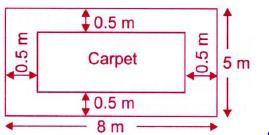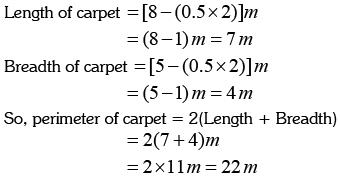QUESTION: 2

### ​What is the area of the given figure?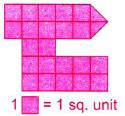Solution:

Number of unit squares = 23
Now, 1 = 1 sq. unit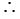Area of figure = 23 square units

QUESTION: 3

### ​The figure is made up of 8 identical rectangles, Find the total area of the shaded figure.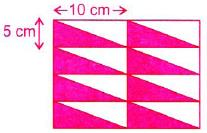Solution:

After rearranging the given figure, we get the new figure.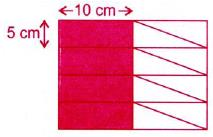Area of 1 small rectangle = 10x5 = 50
sq. cm Number of shaded rectangles ^ 4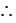Total shaded area = 4x50 = 200 sq. cm

QUESTION: 4

In the given figure, DE = HI = AL = 2 cm and LK = IJ = HG = EF = 5 cm. Find the perimeter of the given figure (in cm).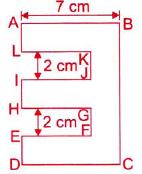Solution:

We have,
BC = AL + KJ + IH + GF + ED
= (2 + 2 + 2 + 2 + 2)cm = 10 cm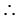Perimeter of the given figure
= (7 +10+7 + 2 +5+ 2 +5+ 2 +5+ 2 +5+ 2)cm 54cm

QUESTION: 5

​ACEG is a square. AB is 1/2 of AC. What is the perimeter of the square?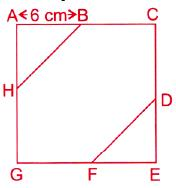Solution:

We have, AB = 1/2 x AC
6cm = 1 x AC = > AC = 6 x 2 = 12 cm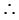Side of square -= 12 cm
So, perimeter of square 12 x 4= 48 cm

QUESTION: 6

What is the area of the shaded figure?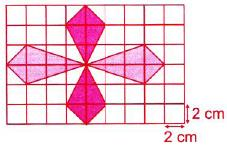Solution:

Area of 1 small square = (2x2)cm2
= 4 cm2
Number of shaded square ^ 14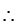Shaded area = 14 x 4 = 56 cm2

QUESTION: 7

Perimeter of any regular figure is _______.

Solution:
QUESTION: 8

The perimeter of a rectangular picture is 160 cm. It is 30 cm wide. How long is it?

Solution:

Perimeter = 160 cm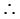2(Length + Breadth) = 160 cm
2(Length + 30 cm) = 160 cm
=> Length +30 cm= 80 cm
=> Length = (80 - 30) cm = 50 cm

QUESTION: 9

​Find the total shaded area of the figure made up of identical squares.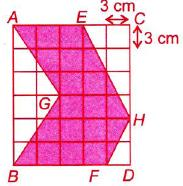Solution:

Area of 1 small square = (3x3)cm2
= 9cm2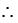Shaded area = 19 x 9 = 171cm2

QUESTION: 10

​Find the perimeter of the given figure.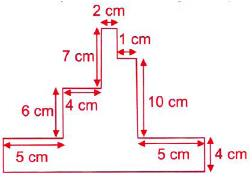Solution:

Perimeter of the given figure
=(2+ 3+1+10+5+ 4 +17+ 4 +5+6+ 4 +7)
cm
= 68cm